# Machine Learning with Python

Get the grades you deserve thru our step by step guide on your MITx MicroMasters Statistics and Data Science Course - Machine Learning with Python: from Linear Models to Deep Learning. MITx 6.86x

# Homework 5Convergence of the Value Iteration Algorithm

### For an Markov Decision Process (MDP) with a single state and a single action, we know the following hold:                                                                                                 𝑉𝑖+1 = 𝑅 + 𝛾𝑉𝑖                                                                                                𝑉∗ = 𝑅 + 𝛾𝑉∗Working with these equations, we can conclude that after each iteration, the difference between the estimate and the optimal value of V decreases by a factor of ? (Enter your answer in terms of  𝛾)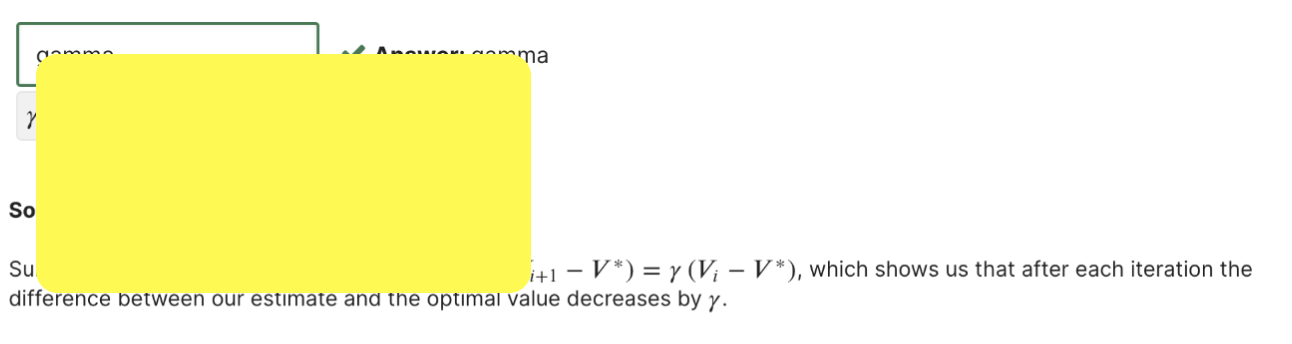# Homework 4K-means and K-medoids

### Assume we have a 2D dataset consisting of . We wish to do k-means and k-medoids. We initialize the cluster centers with (-5, 2 ), ( 0, -6 ).For this small dataset, in choosing between two equally valid exemplars for a cluster in k-medoids, choose them with priorityin the order given above (i.e. all other things being equal, you would choose (0, −6) as a center over (−5, 2)).For the following scenarios, give the clusters and cluster centers after the algorithm converges. Enter the coordinate of eachcluster center as a square-bracketed list (e.g. [0, 0]); enter each cluster's members in a similar format, separated bysemicolons (e.g. [1, 2]; [3, 4]).Clustering 1K-medoids algorithm with l1 norm.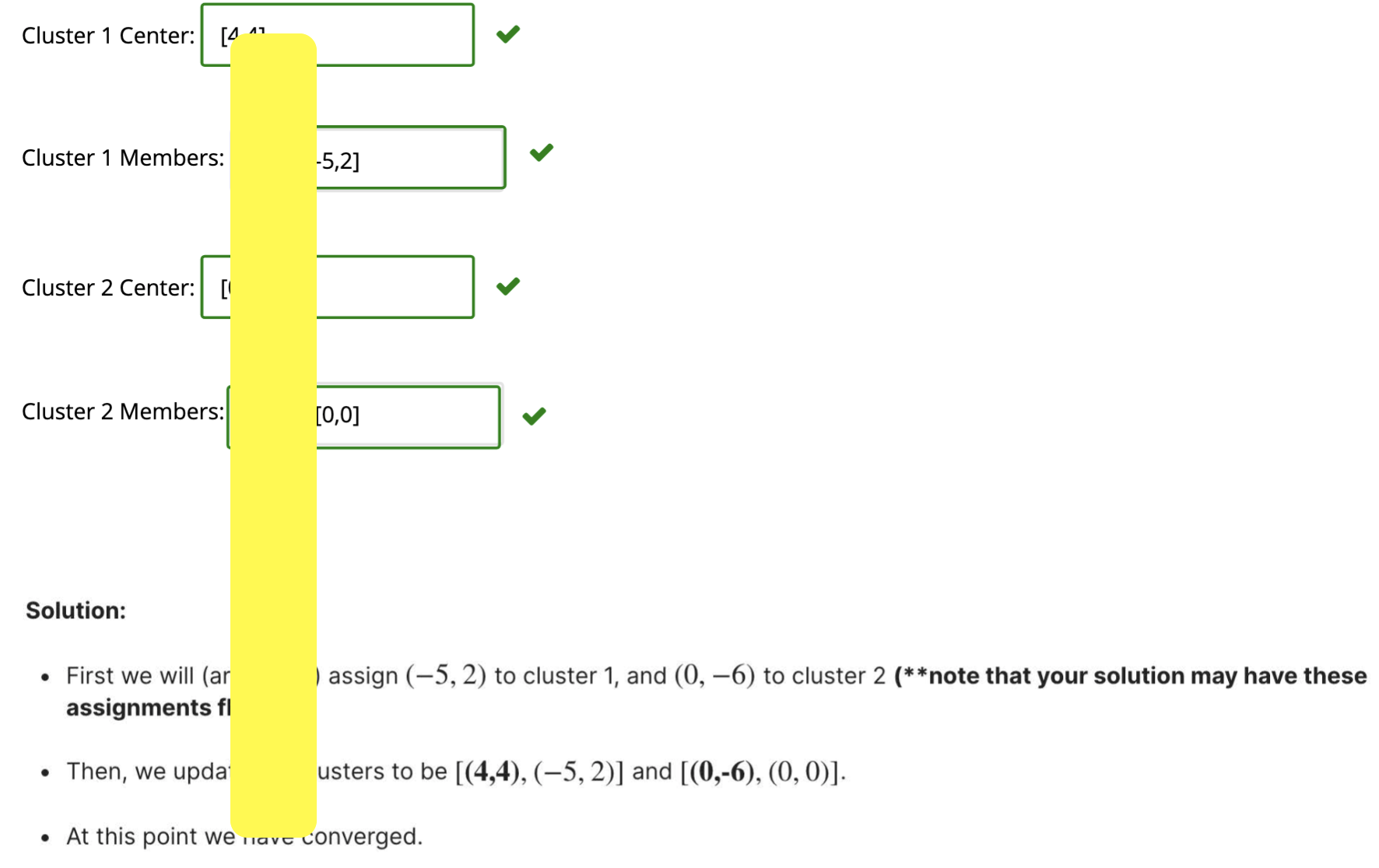## Midterm Exam 1

### Stochastic gradient descent (SGD) is a simple but widely applicable optimization technique. For example, we can use it to train a Support Vector Machine. The objective function in this case is given by: J(θ)=[1/n ∑_(i=1)^n▒  Loss_h⁡(y^((i)) θ⋅x^((i)) )]+λ/2∥θ∥^2 where Loss_h⁡(z)=max{0,1-z} is the hinge loss function, (x^((i)),y^((i)) ) with for i=1,…n are the training examples, with y^((i))∈{1,-1} being the label for the vector x^((i)). For simplicity, we ignore the offset parameter θ_0 in all problems on this page. The stochastic gradient update rule involves the gradient ∇_θ Loss_h⁡(y^((i)) θ⋅x^((i)) ) of Loss_h⁡(y^((i)) θ⋅x^((i)) ) with respect to θ Hint:Recall that for a k-dimensional vector θ=[■(θ_1&θ_2&⋯&θ_k )]^T, the gradient of f(θ) w.r.t. θ is ├ ∇_θ f(θ)=[■(∂f/(∂θ_1 )&∂f/(∂θ_2 )&⋯&∂f/(∂θ_k ))]^T.) Find ∇_θ Loss_h⁡(yθ⋅x) in terms of x. (Enter lambda for λ, y for y and x for the vector x. Use * for multiplication between scalars and vectors, or for dot products between vectors. Use o for the zero vector.)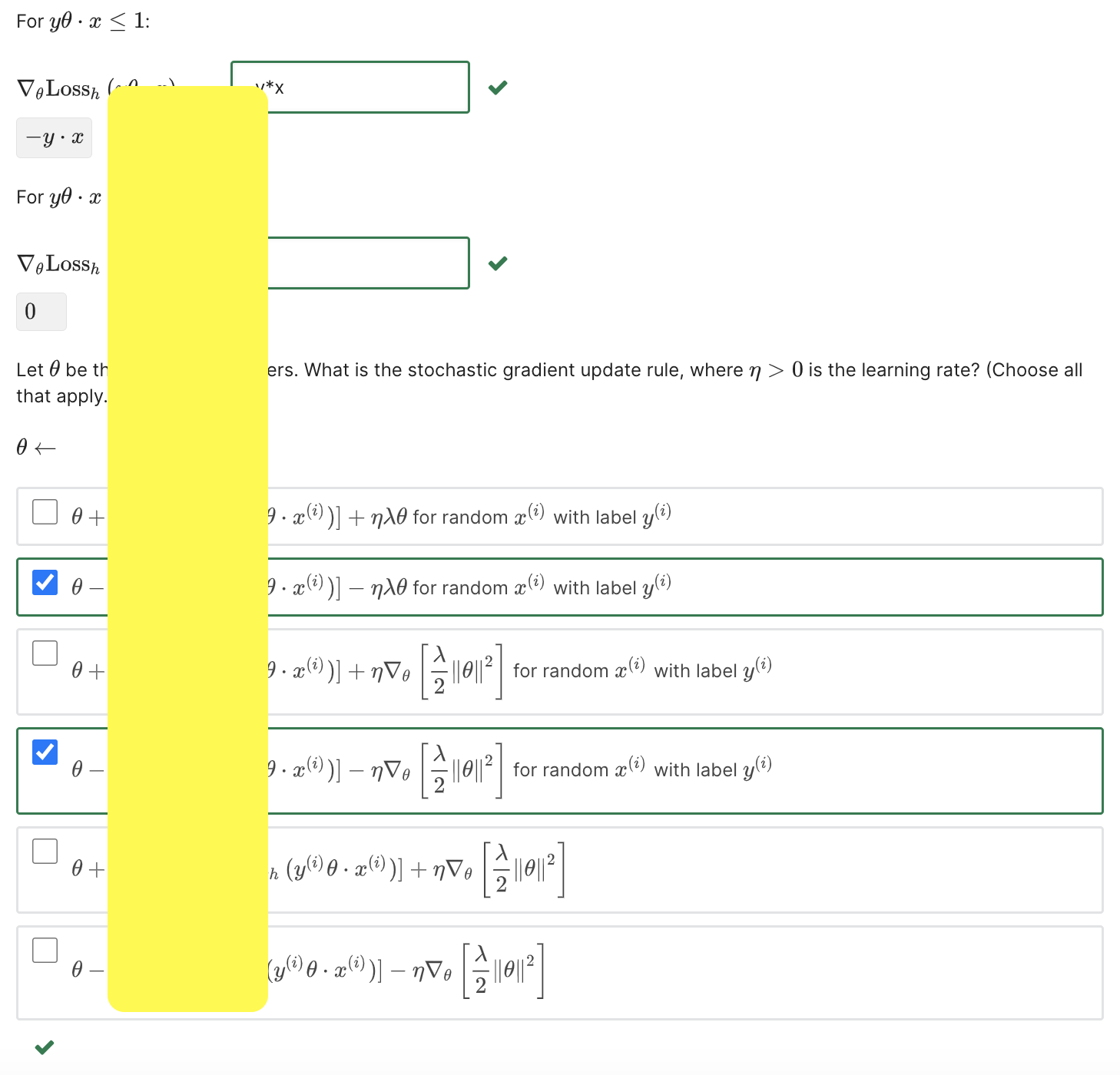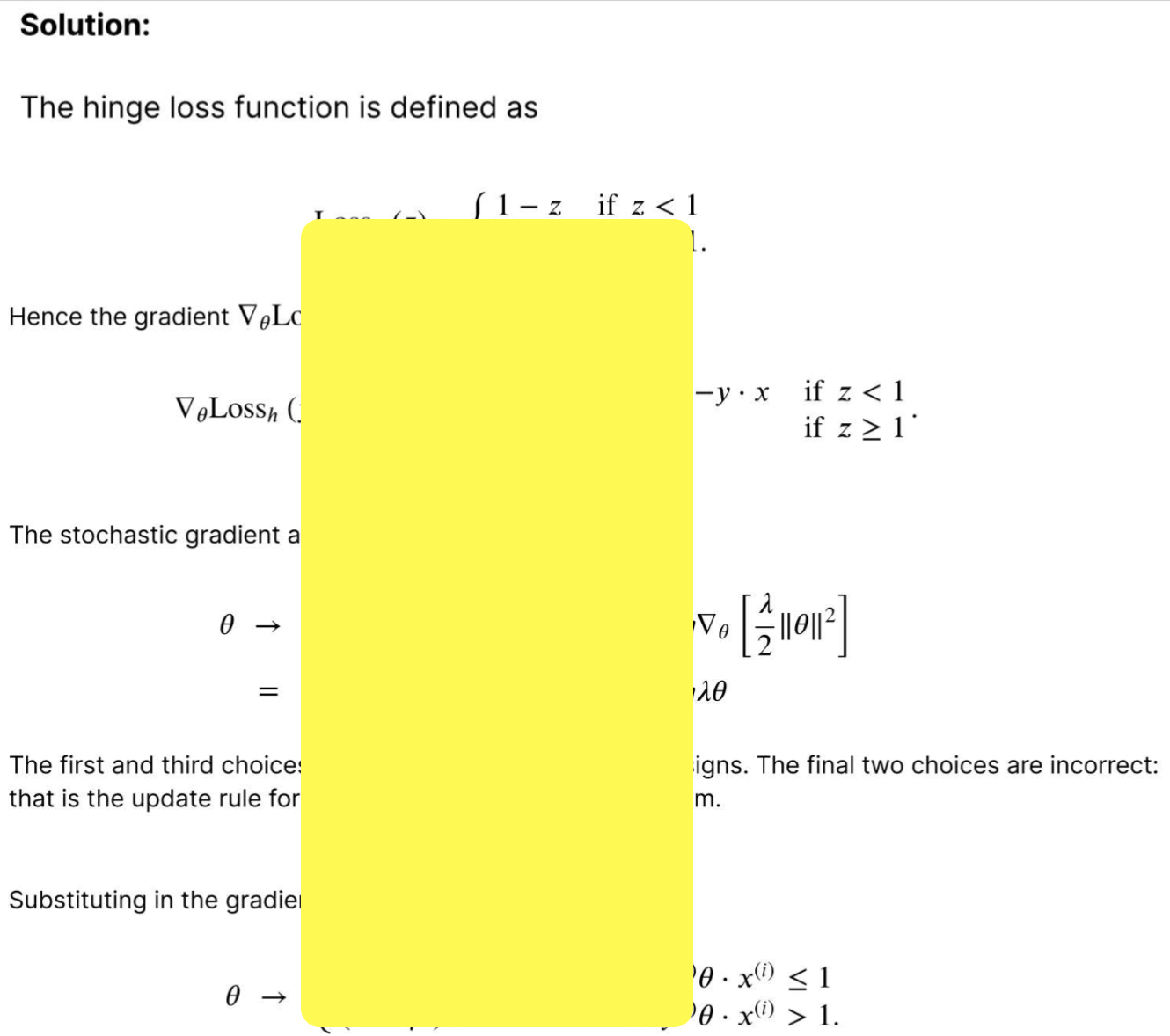## Homework 3Neural Networks

### Feed Forward StepConsider the input 𝑥1 = 3, 𝑥2 = 14. What is the final output (𝑜1, 𝑜2)  of the network ?Important: Numerical outputs from the softmax function are sometimes extremely close to 0 or 1. We recommend you enter you answer as a mathematical expression, such as e∧2+1. If you choose to enter your answers as a decimal, you must enter the decimal accurate to at least 9 decimal places .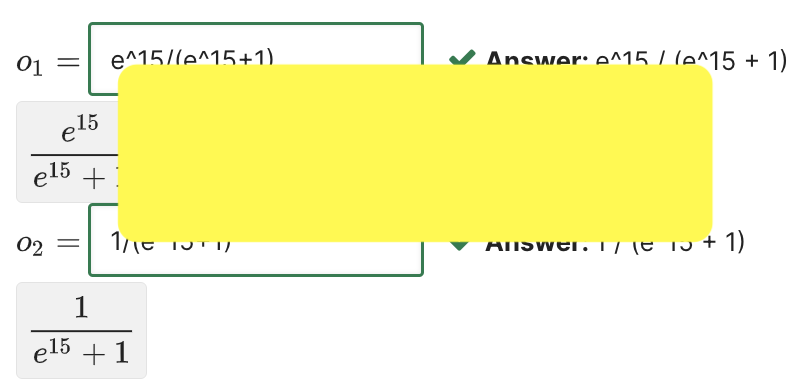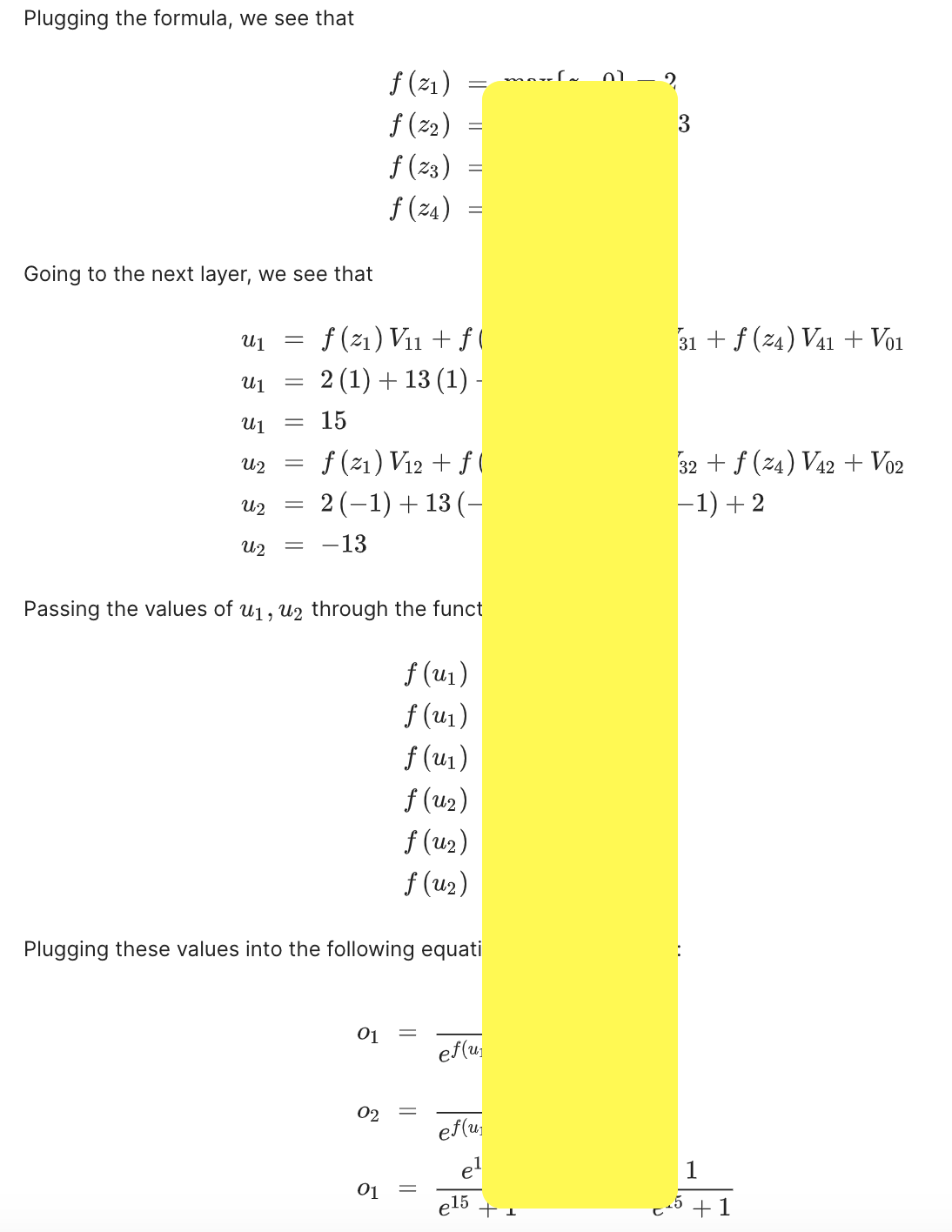# Homework 22. Feature Vectors Transformation

### Consider a sequence of -dimensional data points x(1), x(2), ...., and a sequence of m-dimensional feature vectors, z(1), z(2), ...., extracted from the x's by a linear transformation, z(i) = Ax(i). If m is much smaller than n you might expect that it would be easier to learn in the lower dimensional feature space in the original data space. 2. (a) 1/1 point (graded) Suppose 𝑛 = 6, 𝑚 = 2, 𝑧1 is the average of the elements of 𝑥 and 𝑧2 is the average of the first three elements of 𝑥 minus the average of fourth through sixth elements of 𝑥. Determine ANote: Enter 𝐴 in a list format: [[A11,....A16] [A21,....A26]]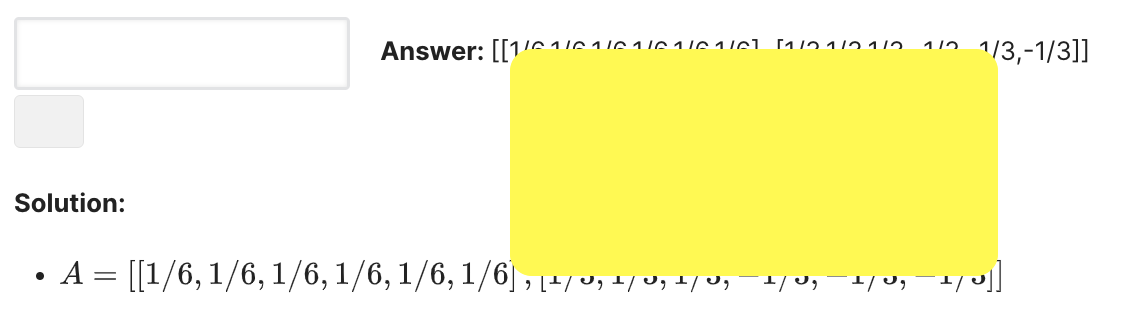# Homework 1Perceptron Mistakes

### In this problem, we will investigate the perceptron algorithm with different iteration ordering.Consider applying the perceptron algorithm through the origin based on a small training set containing three points:                               𝑥(1) = [-1,-1 ],                                                                  𝑦(1) = 1                               𝑥(2) = [1, 0 ],                                                                    𝑦(2) = -1                               𝑥(3) = [-1,1.5 ],                                                                𝑦(3) = 1Given that the algorithm starts with 𝜃(0) = 0, the first point that the algorithm sees is always considered a mistake. The The algorithm starts with some data point and then cycles through the data (in order) until it makes no further mistakes.1. (a) How many mistakes does the algorithm make until convergence if the algorithm starts with data point 𝑥(1)  mistakes does the algorithm make if it starts with data point 𝑥 (2)? Also provide the progression of the separating plane as the algorithm cycles in the following list format: [[𝜃1(1), 𝜃2(1)] , ... , [ 𝜃1(N) 𝜃2(N)]], where the superscript denotes different 𝜃 as the separating plane progresses. For example, if 𝜃 progress from [ 0,0] (initialization) to [1,2] to [3, -2], you should enter [[1,2] , [3, -2]]Please enter the number of mistakes of Perceptron algorithm if the algorithm starts with  𝑥(1) .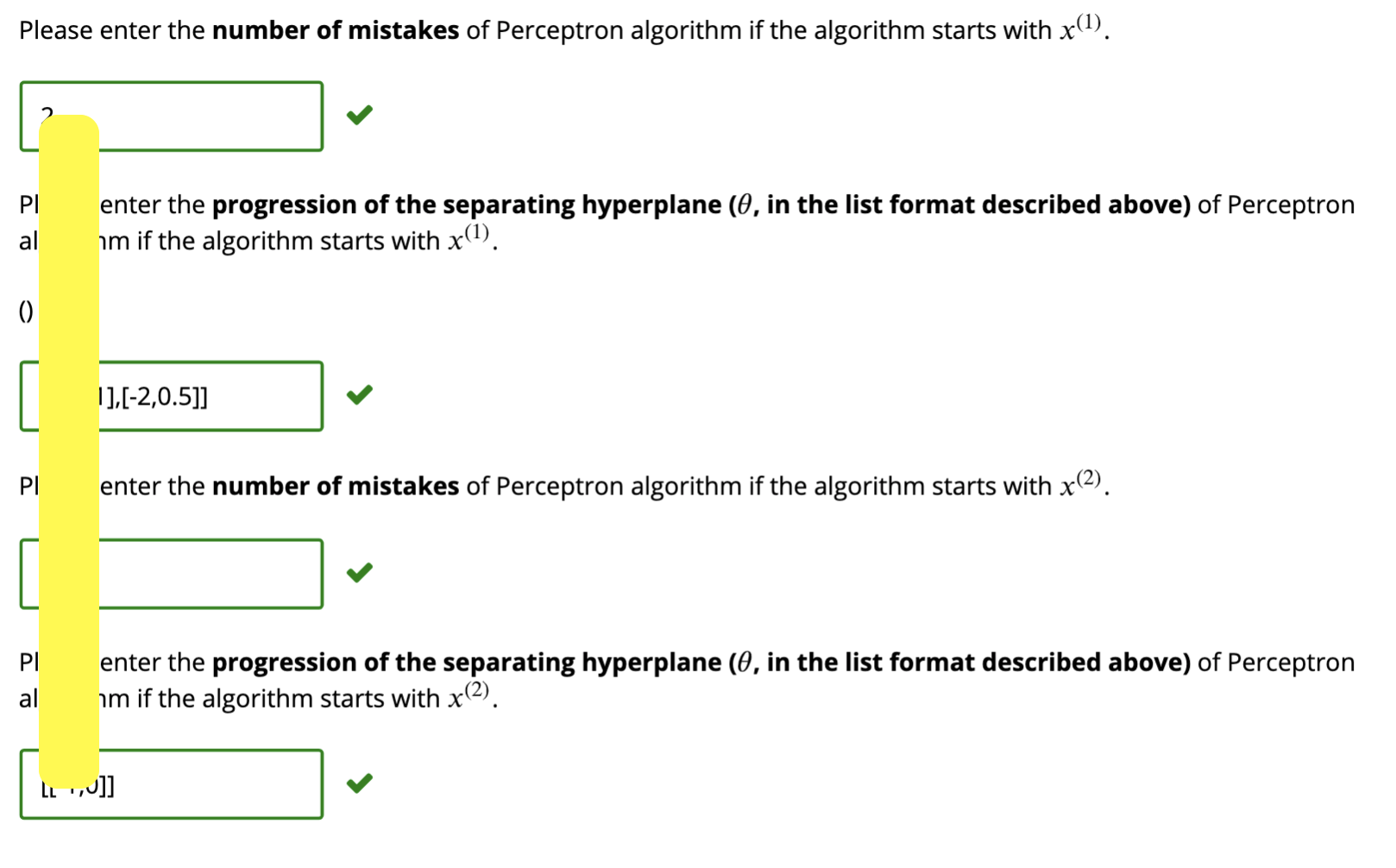This advanced Machine Learning with Python course dives into the basics of machine learning using an approachable, and well-known, programming language. You'll learn about Supervised vs Unsupervised Learning, look into how Statistical Modeling relates to Machine Learning, and do a comparison of each. It combines the approaches of IBM machine learning with python and EDX machine learning with python to offer a practical machine learning with python course that prepares students for complex areas such as pattern recognition.
Pattern recognition is the use of machine learning algorithms to identify patterns. It classifies data based on statistical information or knowledge gained from patterns and their representation. The field of pattern recognition has undergone substantial development over the years and is a form of supervised learning. The main difference between supervised and unsupervised learning: Labeled data. The main distinction between the two approaches is the use of labeled datasets. To put it simply, supervised learning uses labeled input and output data, while an unsupervised learning algorithm does not.
This advanced Machine Learning with Python course dives into the basics of machine learning using an approachable, and well-known, programming language. You'll learn about Supervised vs Unsupervised Learning, look into how Statistical Modeling relates to Machine Learning, and do a comparison of each. It combines the approaches of IBM machine learning with python and EDX machine learning with python to offer a practical machine learning with python course that prepares students for complex areas such as pattern recognition.
Pattern recognition is the use of machine learning algorithms to identify patterns. It classifies data based on statistical information or knowledge gained from patterns and their representation. The field of pattern recognition has undergone substantial development over the years and is a form of supervised learning. The main difference between supervised and unsupervised learning: Labeled data. The main distinction between the two approaches is the use of labeled datasets. To put it simply, supervised learning uses labeled input and output data, while an unsupervised learning algorithm does not.

# Sign up now and get more than 50% off the rack discount!

We can't tell you how we are your best tutor and the answer key to your exams and studies; however, it does have value beyond scoring in your MITx work.
In fact, if you want to get the credentials and not waste your monies for MIT's admission into the SCM program, and have the best learning experience possible, then, you need to use theexamhelper to its full potential. And that applies to the materials as well as supplemental materials – wherever theexamhelper's Solution Key that has explanations and solutions.
What Are the Benefits of Using theexamhelper's Solution Key?

There are 3 main benefits from following this process for completing and reviewing your work.
• Enhanced Understanding of the Concepts Covered
• Improved Self-teaching Skills
• Get high scores for your exams
• Become a Super Learner
• Get admitted into MIT's Masters in Applied Science in Supply Chain Management in MIT

Learners
Solutions
Hrs/Weekly
Success
Special offer

# For a limited time!

Why wait? Pay now or pay later, get the same solutions!
Exclusive
Deal
50% OFF